SLVSA05B August   2009  – August 2015

PRODUCTION DATA.

1. Features
2. Applications
3. Description
4. Revision History
5. Pin Configuration and Functions
6. Specifications
7. Detailed Description
1. 7.1 Overview
2. 7.2 Functional Block Diagram
3. 7.3 Feature Description
4. 7.4 Device Functional Modes
8. Application and Implementation
1. 8.1 Application Information
2. 8.2 Typical Applications
1. 8.2.1 3.3-V to 12-V Boost Converter With PFM Mode at Light Load
1. 8.2.1.1 Design Requirements
2. 8.2.1.2 Detailed Design Procedure
3. 8.2.1.3 Application Curves
2. 8.2.2 3.3-V to 12-V Boost Converter With Forced PWM Mode at Light Load
3. 8.3 System Examples
9. Power Supply Recommendations
10. 10Layout
11. 11Device and Documentation Support
12. 12Mechanical, Packaging, and Orderable Information

• DRC|10
• DRC|10

## 8 Application and Implementation

NOTE

Information in the following applications sections is not part of the TI component specification, and TI does not warrant its accuracy or completeness. TI’s customers are responsible for determining suitability of components for their purposes. Customers should validate and test their design implementation to confirm system functionality.

### 8.1 Application Information

The TPS61086 is designed for output voltages up to 18.5 V with a switch peak current limit of 2.0 A minimum. The device, which operates in a current mode scheme with quasi-constant frequency, is externally compensated for maximum flexibility and stability. The switching frequency is fixed to 1.2 MHz and the minimum input voltage is 2.3 V. To limit the inrush current at start-up, a soft-start pin is available.

TPS61086 boost converter's novel topology using adaptive off-time provides superior load and line transient responses and operates also over a wider range of applications than conventional converters.

### 8.2 Typical Applications

#### 8.2.1 3.3-V to 12-V Boost Converter With PFM Mode at Light Load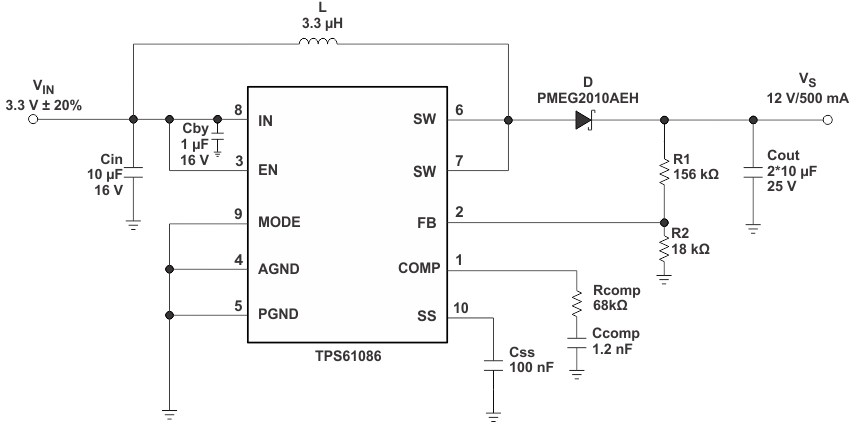Figure 7. Typical Application, 3.3 V to 12 V (PFM Mode)

#### 8.2.1.1 Design Requirements

For this example, the design parameters are listed in Table 2.

### Table 2. Design Parameters

DESIGN PARAMETERS EXAMPLE VALUES
Input Voltage 3.3 V ± 20%
Output Voltage 12 V
Output Current 500 mA
Operation Mode at Light Load PFM

#### 8.2.1.2 Detailed Design Procedure

The first step in the design procedure is to verify that the maximum possible output current of the boost converter supports the specific application requirements. A simple approach is to estimate the converter efficiency, by taking the efficiency numbers from the provided efficiency curves or to use a worst case assumption for the expected efficiency, for example, 90%.

1. Duty cycle, D:
2. Equation 1.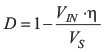3. Maximum output current, Iout(max):
4. Equation 2.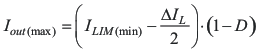5. Peak switch current in application, Iswpeak:
6. Equation 3.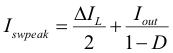with the inductor peak-to-peak ripple current, ΔIL

Equation 4.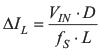where

• VIN is Minimum input voltage.
• VS is Output voltage.
• ILIM(min) is Converter switch current limit (minimum switch current limit = 2.0 A).
• fS is Converter switching frequency (typically 1.2 MHz).
• L is Selected inductor value.
• η is Estimated converter efficiency (please use the number from the efficiency plots or 90% as an estimation.

The peak switch current is the steady-state peak switch current that the integrated switch, inductor and external Schottky diode has to be able to handle. The calculation must be done for the minimum input voltage where the peak switch current is the highest.

#### 8.2.1.2.1 Inductor Selection

The TPS61086 is designed to work with a wide range of inductors. The main parameter for the inductor selection is the saturation current of the inductor which should be higher than the peak switch current as calculated in the Detailed Design Procedure section with additional margin to cover for heavy load transients. An alternative, more conservative, is to choose an inductor with a saturation current at least as high as the maximum switch current limit of 3.2 A. The other important parameter is the inductor DC resistance. Usually the lower the DC resistance the higher the efficiency.

NOTE

The inductor DC resistance is not the only parameter determining the efficiency. Especially for a boost converter where the inductor is the energy storage element, the type and core material of the inductor influences the efficiency as well.

Usually an inductor with a larger form factor gives higher efficiency. The efficiency difference between different inductors can vary between 2% to 10%. For the TPS61086, inductor values between 3 μH and 6 μH are a good choice. Possible inductors are shown in Table 3.

Typically, TI recommends that the inductor current ripple is below 35% of the average inductor current. The following equation can therefore be used to calculate the inductor value, L:

Equation 5.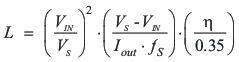where

• VIN is Minimum input voltage.
• VS is Output voltage.
• Iout is Maximum output current in the application.
• fS is Converter switching frequency (typically 1.2 MHz).
• η is Estimated converter efficiency (please use the number from the efficiency plots or 90% as an estimation.

### Table 3. Inductor Selection

L
(μH)
SUPPLIER COMPONENT CODE SIZE
(L×W×H mm)
DCR TYP
(mΩ)
Isat (A)
3.3 Sumida CDH38D09 4 x 4 x 1 240 1.25
4.7 Sumida CDPH36D13 5 x 5 x 1.5 155 1.36
3.3 Sumida CDPH4D19F 5.2 x 5.2 x 2 33 1.5
3.3 Sumida CDRH6D12 6.7 x 6.7 x 1.5 62 2.2
4.7 Würth Elektronik 7447785004 5.9 x 6.2 x 3.3 60 2.5
5 Coilcraft MSS7341 7.3 x 7.3 x 4.1 24 2.9

#### 8.2.1.2.2 Rectifier Diode Selection

To achieve high efficiency a Schottky type should be used for the rectifier diode. The reverse voltage rating should be higher than the maximum output voltage of the converter. The averaged rectified forward current Iavg, the Schottky diode needs to be rated for, is equal to the output current Iout:

Equation 6.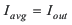Usually a Schottky diode with 1-A maximum average rectified forward current rating is sufficient for most applications. The Schottky rectifier can be selected with lower forward current capability depending on the output current Iout but has to be able to dissipate the power. The dissipated power, PD, is the average rectified forward current times the diode forward voltage, Vforward.

Equation 7.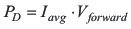Typically the diode should be able to dissipate around 500 mW depending on the load current and forward voltage.

### Table 4. Rectifier Diode Selection

CURRENT RATING Iavg Vr Vforward/Iavg SUPPLIER COMPONENT CODE PACKAGE TYPE
750 mA 20 V 0.425 V / 1 A Fairchild Semiconductor FYV0704S SOT 23
1 A 20 V 0.39 V / 1 A NXP PMEG2010AEH SOD 123
1 A 20 V 0.5 V / 1 A Vishay Semiconductor SS12 SMA
1 A 20 V 0.44 V / 1 A Vishay Semiconductor MSS1P2L Âµ -SMP
2 A 20 V 0.44 V / 2 A Vishay Semiconductor SL22 SMB

#### 8.2.1.2.3 Setting the Output Voltage

The output voltage is set by an external resistor divider. Typically, a minimum current of 50 μA flowing through the feedback divider gives good accuracy and noise covering. A standard low side resistor of 18 kΩ is typically selected. The resistors are then calculated as:

Equation 8.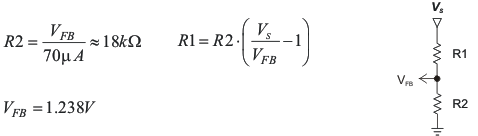#### 8.2.1.2.4 Compensation (COMP)

The regulator loop can be compensated by adjusting the external components connected to the COMP pin. The COMP pin is the output of the internal transconductance error amplifier.

Standard values of RCOMP = 16 kΩ and CCOMP = 2.7 nF will work for the majority of the applications.

Please refer to Table 5 for dedicated compensation networks giving an improved load transient response. The following equations can be used to calculate RCOMP and CCOMP:

Equation 9.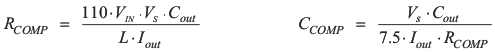where

• VIN is Minimum input voltage.
• VS is Output voltage.
• Cout is Output capacitance.
• L is Inductor value, for example, 3.3 μH or 4.7 μH.
• Iout is Maximum output current in the application.

Make sure that RCOMP < 120 kΩ and CCOMP> 820 pF, independent of the results of the above formulas.

### Table 5. Recommended Compensation Network Values at High/Low Frequency

L VS VIN ± 20% RCOMP CCOMP
3.3 μH 15 V 5 V 100 kΩ 820 pF
3.3 V 91 kΩ 1.2 nF
12 V 5 V 68 kΩ 820 pF
3.3 V 68 kΩ 1.2 nF
9 V 5 V 39 kΩ 820 pF
3.3 V 39 kΩ 1.2 nF

Table 5 gives conservative RCOMP and CCOMP values for certain inductors, input and output voltages providing a very stable system. For a faster response time, a higher RCOMP value can be used to enlarge the bandwidth, as well as a slightly lower value of CCOMP to keep enough phase margin. These adjustments should be performed in parallel with the load transient response monitoring of TPS61086.

#### 8.2.1.2.5 Input Capacitor Selection

For good input voltage filtering low-ESR ceramic capacitors are recommended. TPS61086 has an analog input IN. Therefore, a 1-μF bypass is highly recommended as close as possible to the IC from IN to GND.

One 10-μF ceramic input capacitors are sufficient for most of the applications. For better input voltage filtering this value can be increased. Refer to Table 6 and typical applications for input capacitor recommendation

#### 8.2.1.2.6 Output Capacitor Selection

For best output voltage filtering a low-ESR output capacitor like ceramic capcaitor is recommended. Two to four 10-μF ceramic output capacitors (or two 22 μF) work for most of the applications. Higher capacitor values can be used to improve the load transient response. Refer to Table 6 for the selection of the output capacitor.

### Table 6. Rectifier Input and Output Capacitor Selection

CAPACITOR/SIZE VOLTAGE RATING SUPPLIER COMPONENT CODE
CIN 22 μF/1206 16 V Taiyo Yuden EMK316 BJ 226ML
IN bypass 1 μF/0603 16 V Taiyo Yuden EMK107 BJ 105KA
COUT 10 μF/1206 25 V Taiyo Yuden TMK316 BJ 106KL

To calculate the output voltage ripple, the following equation can be used:

Equation 10.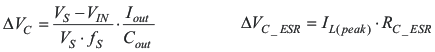where

• ΔVC is Output voltage ripple dependent on output capacitance,output current and switching frequency.
• VS is Output voltage.
• VIN is Minimum input voltage of boost converter.
• fS is Converter switching frequency (typically 1.2 MHz).
• Iout is Output capacitance.
• ΔVC_ESR is Output voltage ripple due to output capacitors ESR (equivalent series resistance).
• ISWPEAK is Inductor peak switch current in the application.
• RC_ESR is Output capacitors equivalent series resistance (ESR).

ΔVC_ESR can be neglected in many cases since ceramic capacitors provide very low ESR.

#### 8.2.1.3 Application Curves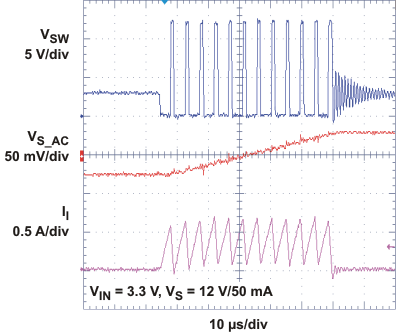Figure 8. PFM Mode Switching Pulse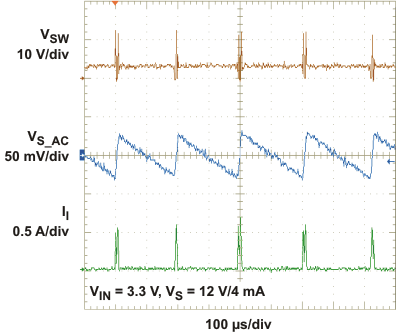Figure 10. PFM Mode - Light Load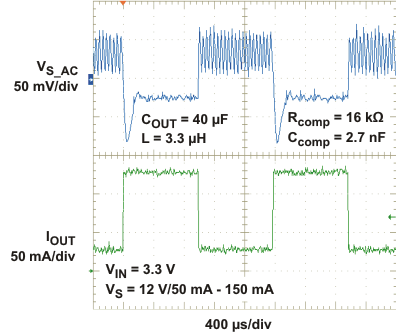Figure 12. Load Transient Response PFM Mode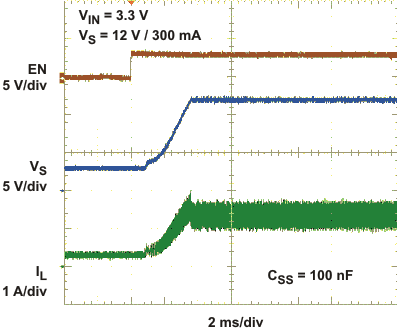Figure 14. Soft-Start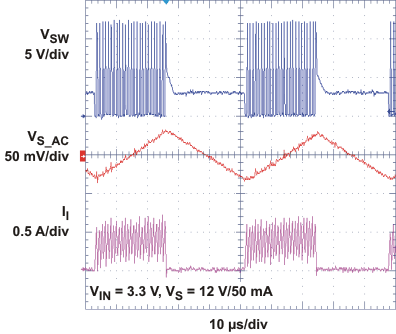Figure 9. PFM Mode Switching Pulses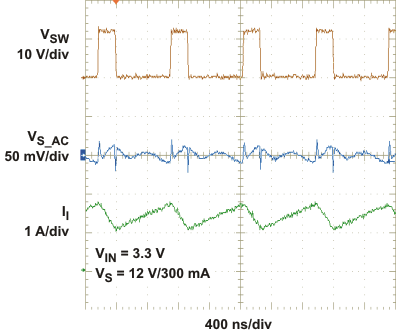Figure 11. Forced PWM / PFM Mode - Heavy Load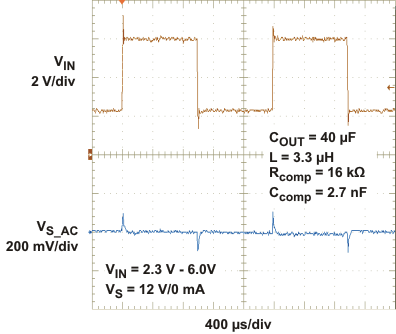Figure 13. Line Transient Response Light Load

#### 8.2.2 3.3-V to 12-V Boost Converter With Forced PWM Mode at Light Load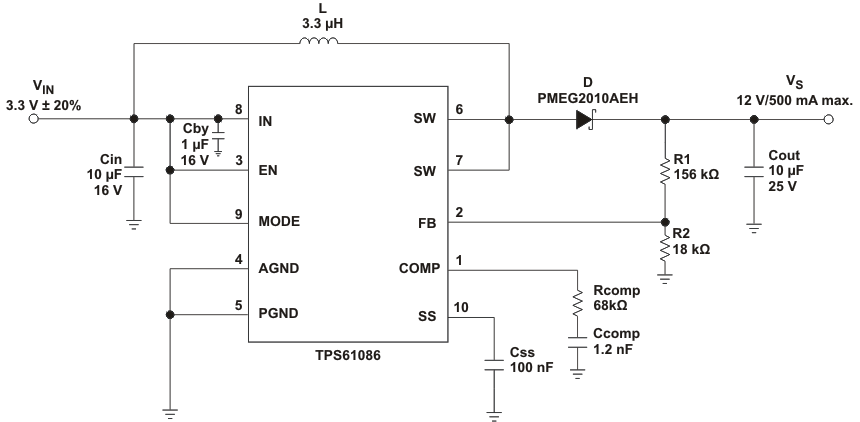Figure 15. Typical Application, 3.3 V to 12 V (Force PWM Mode)

#### 8.2.2.1 Design Requirements

For this example, the design parameters are listed in Table 7.

### Table 7. Design Parameters

DESIGN PARAMETERS EXAMPLE VALUES
Input Voltage 3.3 V ± 20%
Output Voltage 12 V
Output Current 500 mA
Operation Mode at Light Load Forced PWM

#### 8.2.2.2 Detailed Design Procedure

Refer to Detailed Design Procedure in the 3.3-V to 12-V Boost Converter With PFM Mode at Light Load section.

#### 8.2.2.3 Application CurvesFigure 16. Forced PWM Mode - Light Load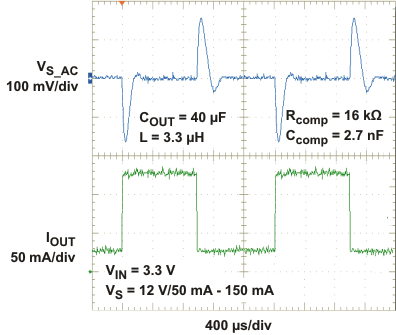Figure 18. Load Transient Response Force PWM ModeFigure 17. Forced PWM / PFM Mode - Heavy Load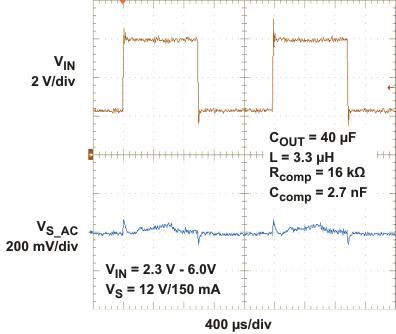Figure 19. Line Transient Response Heavy Load

### 8.3 System Examples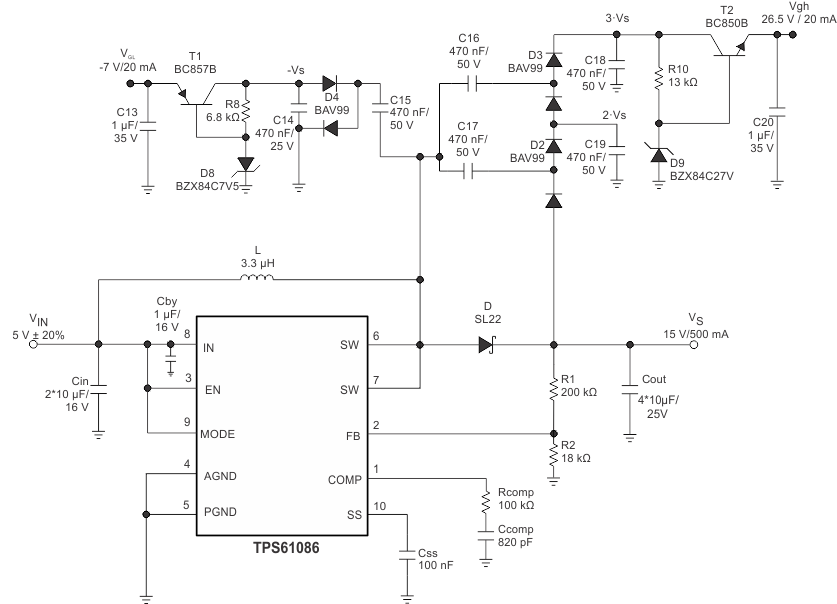Figure 20. Typical Application 5 V to 15 V (Force PWM Mode) for TFT LCD With External Charge Pumps (VGH, VGL)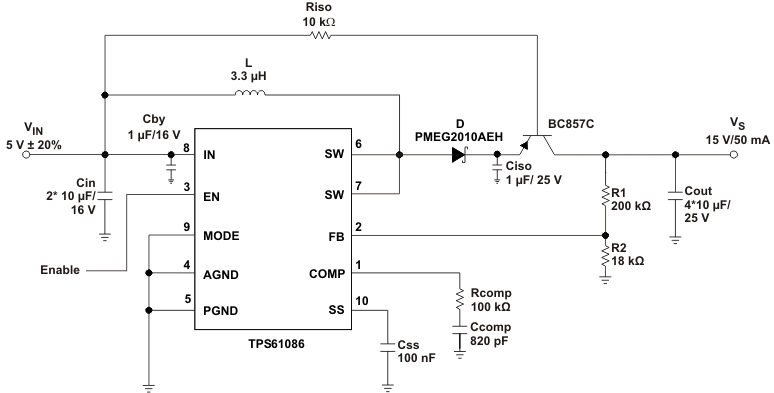Figure 21. Typical Application With External Load Disconnect SwitchFigure 22. Typical Application, 3.3 V to 15 V (PFM Mode) With Overvoltage ProtectionFigure 23. Simple Application (3.3-V Input Voltage - Forced PWM Mode) for wLED Supply (3S3P) (With Optional Clamping Zener Diode)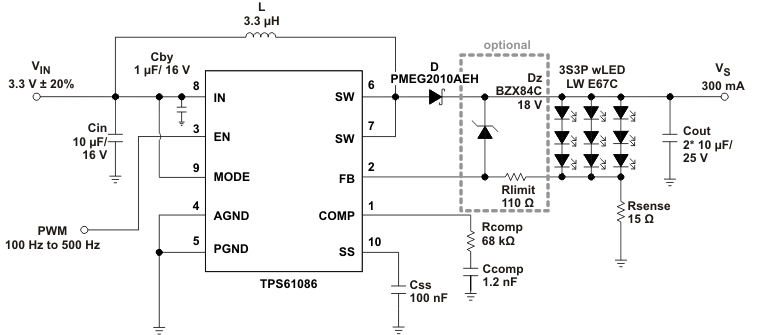Figure 24. Simple Application (3.3-V Input Voltage - Forced PWM Mode) for wLED Supply (3S3P) With Adjustable Brightness Control Using a PWM Signal on the Enable Pin (With Optional Clamping Zener Diode)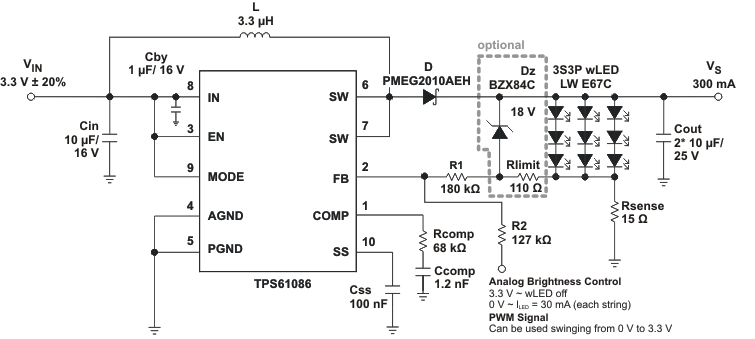Figure 25. Simple Application (3.3-V Input Voltage - Forced PWM Mode) for wLED Supply (3S3P) With Adjustable Brightness Control Using an Analog Signal on the Feedback Pin (With Optional Clamping Zener Diode)